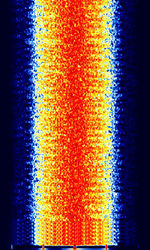# KG-STV

KG-STVFrequencies 3.733 MHz,7.173 MHz,14.233 MHz
Frequency Range 3.733 MHz - 14.233 MHz
Mode USB
Modulation 4FSK MSK
ACF Header: variable, Trailer: 142.5 ms
Emission Designator
Bandwidth 500 Hz,2.5 kHz
Location Worldwide
Short Description KG-STV is an image transmission mode developed by JJ0OBZ in Japan.
I/Q Raw Recording
Audio Sample

KG-STV is an image transmission mode developed by JJ0OBZ in Japan. The mode transmits one 16x16 compressed jpeg block at a time, so that partial transmissions can still reveal the image with missing holes. You can see each block as it's received being reconstructed. The software allows the receiver to send a repeat request in case there are received blocks that have errors. In addition to images, users can send and receive short text messages to each other with this mode.

KG-STV has two modulation modes. MSKMinimum-Shift Keying (When Shift/Bd = 0.5. It is impossible to get this ratio to be lower than 0.5, hence it is called the 'Minimum' shift.) (Minimum Shift-Keying) and 4LFSK (4-Level Frequency-Shift Keying). The 4LFSK mode transmits twice as fast as MSKMinimum-Shift Keying (When Shift/Bd = 0.5. It is impossible to get this ratio to be lower than 0.5, hence it is called the 'Minimum' shift.), but is more prone to being affected significantly by propagation effects. Most transmissions are done in MSKMinimum-Shift Keying (When Shift/Bd = 0.5. It is impossible to get this ratio to be lower than 0.5, hence it is called the 'Minimum' shift.) mode. Each mode has a choice of convolution encoding or no encoding. The error correction used is Viterbi Encoding (NASA standard K=7 mode)

MSKMinimum-Shift Keying (When Shift/Bd = 0.5. It is impossible to get this ratio to be lower than 0.5, hence it is called the 'Minimum' shift.) uses two frequencies, 1800 HzHertz (Hz), unit of frequency, defined as one cycle per second (1 Hz). for 1 and 1200 HzHertz (Hz), unit of frequency, defined as one cycle per second (1 Hz). for 0. For 4L-FSKFrequency-Shift Keying, '00' 1200 HzHertz (Hz), unit of frequency, defined as one cycle per second (1 Hz).; '01' 1400 HzHertz (Hz), unit of frequency, defined as one cycle per second (1 Hz).; '10' 1600 HzHertz (Hz), unit of frequency, defined as one cycle per second (1 Hz).; '11' 1800 HzHertz (Hz), unit of frequency, defined as one cycle per second (1 Hz)..

All signals operate at 1200 BdBaud (unit symbol Bd) is the unit for symbol rate or modulation rate in symbols per second., and occupy a bandwidth of between 500 HzHertz (Hz), unit of frequency, defined as one cycle per second (1 Hz). to 2500 HzHertz (Hz), unit of frequency, defined as one cycle per second (1 Hz)., depending on signal quality.

## Samples

MSKMinimum-Shift Keying (When Shift/Bd = 0.5. It is impossible to get this ratio to be lower than 0.5, hence it is called the 'Minimum' shift.) MSKMinimum-Shift Keying (When Shift/Bd = 0.5. It is impossible to get this ratio to be lower than 0.5, hence it is called the 'Minimum' shift.) w/with Convolution
Text Transmission:
4LFSK MSKMinimum-Shift Keying (When Shift/Bd = 0.5. It is impossible to get this ratio to be lower than 0.5, hence it is called the 'Minimum' shift.)
Quality comparison:

Original Image:

## Frequencies

KG-STV can be seen on amateur SSTV bands: 3733 kHzKiloHertz (kHz) 10^3 Hz, 7173 kHzKiloHertz (kHz) 10^3 Hz, and 14233 kHzKiloHertz (kHz) 10^3 Hz.

## Decoding Software

Hobby Level Software